# Querying with DAX

Below some DAX queries.

 EVALUATE ‘Dimension’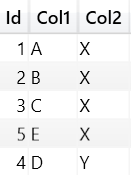EVALUATE ‘Fact’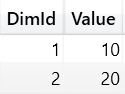EVALUATE ‘Dimension’ ORDER BY ‘Dimension'[Id] START AT 3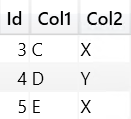EVALUATE CALCULATETABLE(‘Dimension’, ‘Dimension'[Col2]=”X”)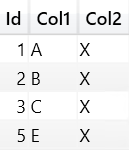EVALUATE ADDCOLUMNS(‘Dimension’, “TotalValue”, [TotalValue])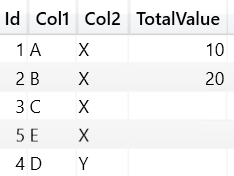EVALUATE SUMMARIZE(‘Dimension’, ‘Dimension'[Col1])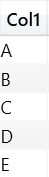EVALUATE SELECTCOLUMNS( ‘Dimension’, “Column 1”,’Dimension'[Col1], “Column 2”,’Dimension'[Col2])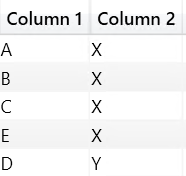EVALUATE SUMMARIZE( ‘Dimension’, ROLLUP(‘Dimension'[Col1]), “TotalValue”, [TotalValue])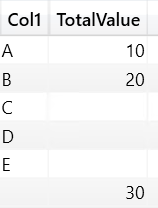EVALUATE CROSSJOIN( ALL(‘Dimension'[Col1]) , ALL(‘Dimension'[Col2]))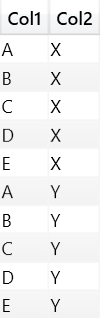EVALUATE GENERATE(‘Dimension’, RELATEDTABLE(‘Fact’))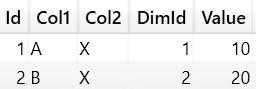EVALUATE ROW(“A”, 1, “B”, 2)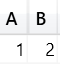EVALUATE TOPN(3,’Dimension’)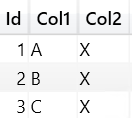EVALUATE UNION(ROW(“A”, 1),ROW(“B”, 2))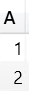EVALUATE INTERSECT(ROW(“A”, 1),ROW(“B”, 1))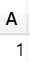EVALUATE SUMMARIZECOLUMNS( ‘Dimension'[Col1], ‘Dimension'[Col2], “TotalValue”, [TotalValue])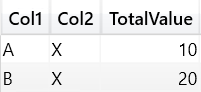EVALUATE GROUPBY( ‘Fact’ ,’Dimension'[id] ,”TotalValue”, SUMX(CURRENTGROUP(), ‘Fact'[Value]))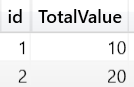WITH MEASURE ‘Fact'[TotalValue1] = SUMX(‘Fact’, ‘Fact'[Value]) SELECT {[TotalValue1]} ON COLUMNS, [Dimension].[Id].MEMBERS ON ROWS FROM [Model]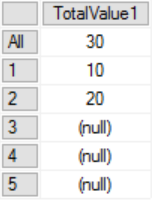DEFINE MEASURE ‘Fact'[TotalValue] = SUMX(‘Fact’, ‘Fact'[Value]) EVALUATE ADDCOLUMNS( SUMMARIZE( ‘Dimension’ ,’Dimension'[Id]) , “TotalValue”, [TotalValue])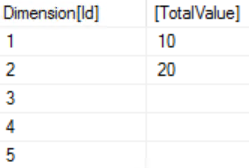# Time Intelligence Functions

The image below shows the output of each Time Intelligence Function when we pass as parameter a column ‘Date’[Date] containing two dates: 2017-12-01 and 2017-12-02.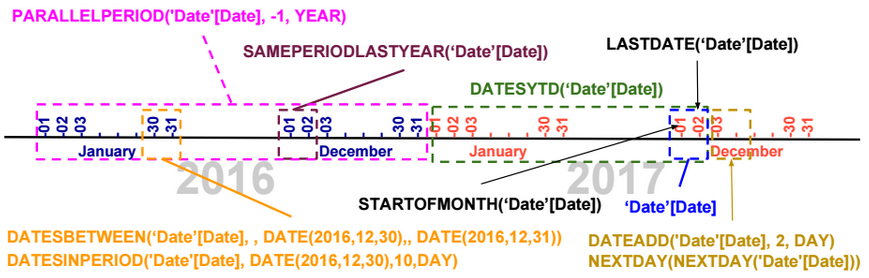Using the CALCULATE function, we can evaluate a measure based on the result of a Time Intelligence function.

CALCULATE([TotalSales], DATESYTD(‘Date’[Date]))

# Last Non Blank Function

LastNonBlank is a DAX function that returns the last value of a column for which an expression is not blank.

The DAX expression below returns the last date the measure Stock is not blank.

LASTNONBLANK(‘Date'[Date], [Stock])

# Calendar Functions

To generate a calendar, we can use the following functions: CALENDAR, CALENDARAUTO.

# Table Functions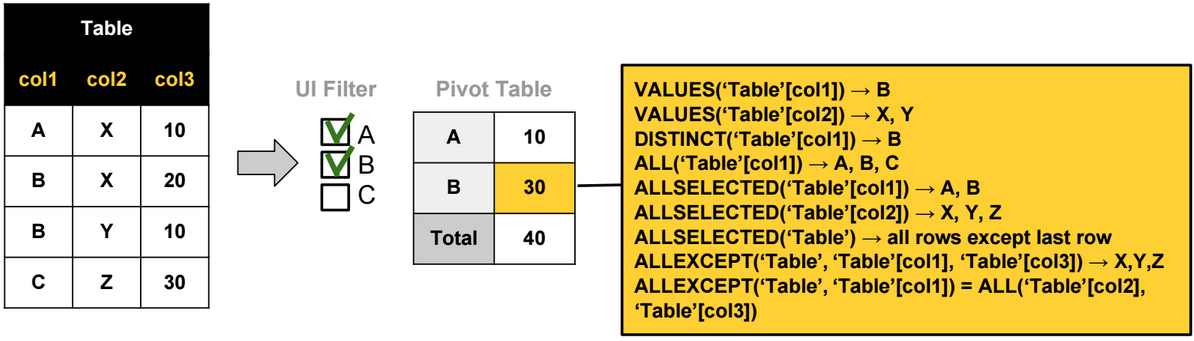The most used Table functions are:

ALL: Ignores any filters that might have been applied on a table or a column.

ALLSELECTED: Ignores any filters that might have been applied on a table or a column, but retains explicit filters (like Excel slicers). (In reality, this function removes all filter contexts generated by context transitions).

DISTINCT: Returns a one-column table that contains the distinct values from the specified column

VALUES: Returns a one-column table that contains the distinct values from the specified table or column.

ALLEXCEPT: Ignores any filters that might have been applied except on the specified columns. For example ALLEXCEPT(‘Table’, Table’[col1]) is equivalent to ALL(‘Table’[col2],‘Table ’[col3]).

ALLNOBLANKROW: Ignores any filters that might have been applied on a table or a column, returns all rows but the blank row, or all distinct values of a column but the blank row.

# Path Functions

Path functions are usually used when defining parent-child hierarchies. Below an example that shows how we can use these functions to create a parent-child hierarchy.

In the table below, we have a parent-child relationship based on two columns: Id and ParentId.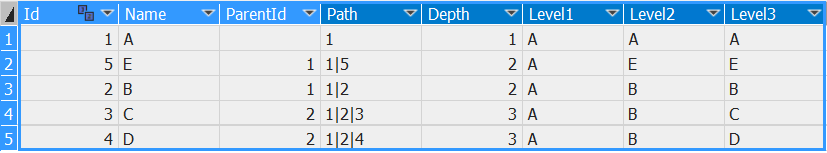The 5 calculated columns on the right are defined using the following DAX expressions:

 Calculated Column DAX Expression Path =PATH([Id],[ParentId]) Depth =PATHLENGTH([Path]) Level1 =IF([Depth] >=1, LOOKUPVALUE([Name],[Id], VALUE(PATHITEM([Path],1,1))), [Name]) Level2 =IF([Depth] >=2, LOOKUPVALUE([Name],[Id], VALUE(PATHITEM([Path],2,1))), [Name]) Level3 =IF([Depth] >=3, LOOKUPVALUE([Name],[Id], VALUE(PATHITEM([Path],3,1))), [Name])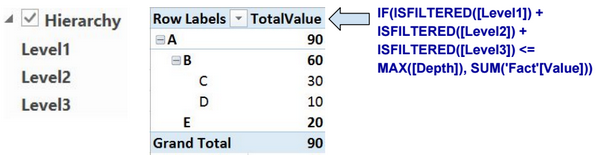We use the columns Level1, Level2, and Level3 to define the parent-child hierarchy.

# LookUpValue Function

LookupValue function retrieves a lookup value from a lookup table.

# Count Functions

COUNT: Count numeric values.

COUNTA: Count rows different than blank.

COUNTBLANK: Count rows equal to blank.

COUNTROWS: COUNTA + COUNTBLANK.

DISTINCTCOUNT: Distinct count including Blank values.

# Other Functions

ISEMPTY

IFERROR, ISERROR

LEN, LEFT, RIGHT, SEARCH, SUBSTITUTE, REPLACE, FORMAT

DIVIDE

SWITCH

ISBLANK, ISTEXT, ISNONTEXT, ISNUMBER

CONCATENATEX

RANKX

ISFILTERED, ISCROSSFILTERED

HASONEVALUE

FLOOR, TRUNC, ROUNDDOWN, CEILING, MROUND, ROUNDUP

YEAR, MONTH, DAY

More details about these functions can be found here: https://msdn.microsoft.com/en-us/library/ee634396.aspx

# Evaluation Contexts

There are 3 types of evaluation contexts.

 Row Context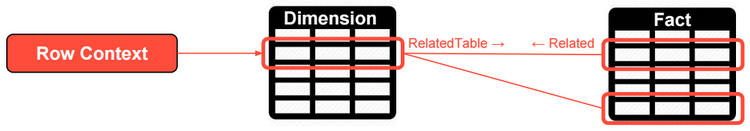Row context includes the values from all columns in a row and related rows. Row contexts doesn’t propagate through relationships (from the one side to the many side of one-to-many relationships). Filter Context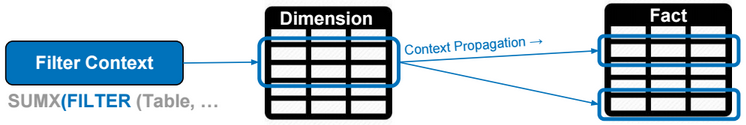Filter context specifies filter constraints on the set of values allowed in a column or a table. Filter context propagates through relationships. Query Context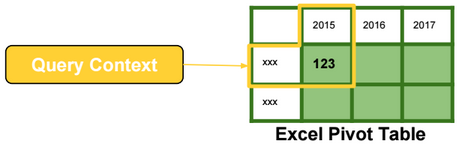Query context is the filtering applied to a cell in the pivot table. The filter context and the query context are almost identical in their effects.

# X Functions

X Functions are iterators. They evaluate expressions by iterating over table rows.

The following DAX expression is evaluated by running a loop over rows of the Sales table.

[TotalSales] = SUMX (Sales, Sales[Quantity] * Sales[Net Price])Rows with values not allowed in the filter/query context are ignored.

# Context Transition

Context transition is the transformation of row contexts into an equivalent filter context.

For example, to evaluate the DAX expression below, we need to iterate over rows in the Date table. For each Date row, we need to evaluate the measure TotalSales, and then calculate the average of daily sales.

AvgDailySales = AverageX(Date, [TotalSales])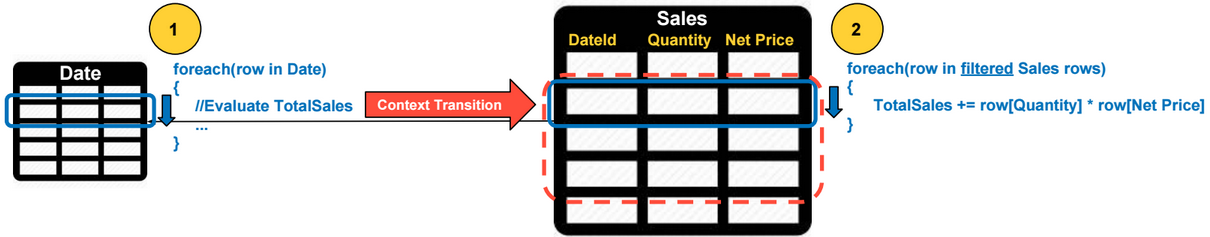For each Date row, a context transition occurs, and values from all columns in a Date row are used as filters when evaluating the TotalSales. Context transition occurs because TotalSales is a measure.

Context transition automatically occurs when the input expression of the X function is a measure, otherwise we need to use the CALCULATE function to perform context transitions like in the following example:

AvgDailySales = AverageX(Date, CALCULATE(SUMX(Sales, Sales[Quantity] * Sales[Net Price])))

# Filter Context Intersection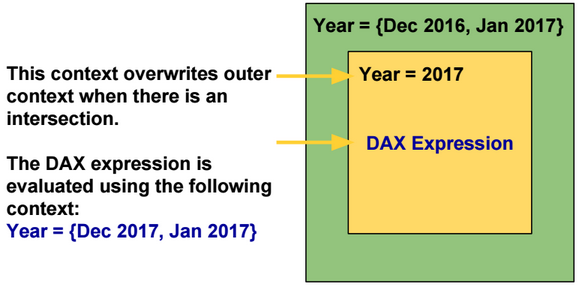By default inner filter contexts overwrite outer filter contexts when there is an intersection.

The KEEPFILTERS function modifies this behavior by using Intersect instead of Overwrite.

 Overwrite Intersect -Outer Filter Context: {Dec 2016, Jan 2017} -Inner Filter Context: {Year = 2017} {Dec 2017, Jan 2017} {Jan 2017}

# Filter Function

Filter function is also an iterator. It filters rows based on a list of input conditions.

# Calculate Function

CALCULATE evaluates an expression in a context that is modified by the specified filters.

The following DAX expressions are equivalent. The filter expression of the calculate function can be a table.

[TotalSales2017] = CALCULATE([TotalSales], ‘Date'[Year] = 2017)

[TotalSales2017] = CALCULATE([TotalSales], FILTER(ALL(‘Date'[Year]), ‘Date'[Year] = 2017)

CALCULATE always performs context transition by transforming row context to filter context. In the DAX expressions above, the row context is empty.

# Context Propagation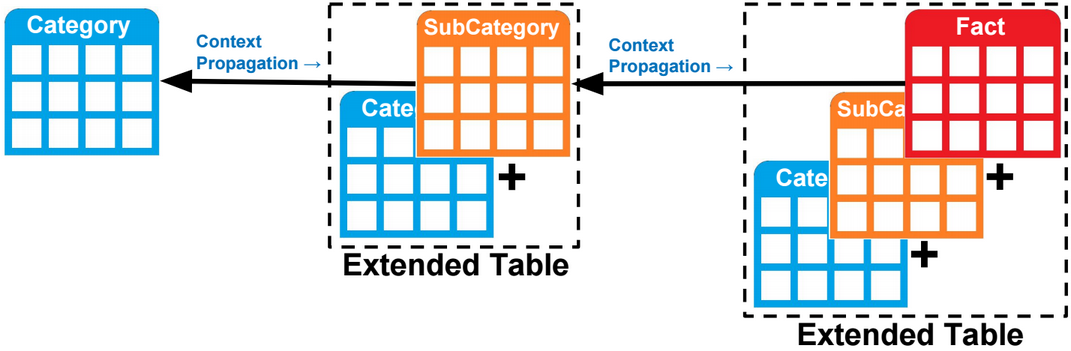Filter context propagates (by default) from the one side to the many side of a one-to-many relationship.

Filter context can also propagates from the many side to the one side of a one-to-many relationship. For example, the DAX expression below counts the number of rows in the category table when one or many sub categories are selected.

CALCULATE(COUNTROWS(‘Category’), ‘SubCategory‘)

In reality, there is no context propagation, but instead, tables are extended to include all columns in related tables, and filtering a column instantly filters all extended tables referencing that column.

To propagate a filter context from the many side to the one side of a one-to-many relationship, we need to use the Calculate function and specify as parameter the many side table.

ALL function removes all filters defined on a table and related tables. To keep filters defined on a related table, we need to specify that related table as a parameter to the calculate function.

CALCULATE(COUNTROWS(‘SubCategory’), ALL(‘SubCategory’), ‘Category’)

# Relationships

Relationships in a model could be one-way or two-way. A relationship is deactivated if it’s causing ambiguity in the model.

The model below shows two paths to propagate contexts from the table on the left to the one on the right. This model is not supported and one of the relationships should be deactivated.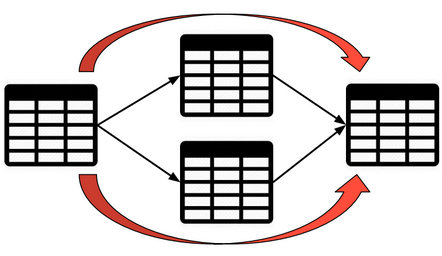To activate and use a relationship when evaluating a DAX expression, we can use the USERELATIONSHIP function.

CALCULATE([TotalSales], USERELATIONSHIP(Sales[OrderDate],Date[Id]))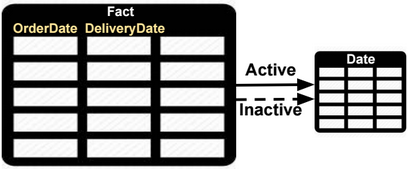To change the filter direction of a relationship, we can use the CROSSFILTER function.

CALCULATE([TotalSales], CROSSFILTER(Sales[OrderDate],Date[Id], BOTH))

# Many-to-many Relationships

Relationships in a model could be one-way or two-way. In a two-way relationship, filter contexts propagate in both directions.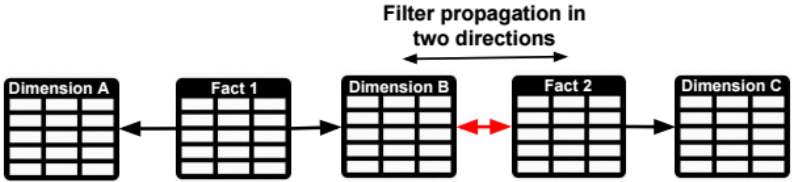If the model doesn’t support two-way relationships, then we need to specify the many-to-many (M2M) fact table as parameter of the Calculate function.

For example, if we want to propagate a filter on Dimension C to Fact1, we only need to specify Fact 2 as parameter of the Calculate function. The extended Fact 2 table includes all columns of related tables (Dimensions B and C), and filtering Fact 2 table also filters Dimension B columns that are in the extended Fact 2 table.

CALCULATE([TotalSales], Fact2)

# Virtual Relationships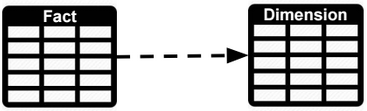We can simulate a relationship between two tables using DAX. For example to calculate the TotalSales for values in a dimension table we can use the following formula:

CALCULATE([TotalSales], FILTER(‘Fact’, CONTAINS(‘Dimension’, ‘Dimension'[Id], ‘Fact'[Id])))

# Calculated Column vs Measure

 Calculated column Consume more memory. The filter context is always empty when defining a Calculated Column. Measure Consume more CPU. The filter context is automatically applied on measures.